## Appendix H - Chart Indicators

 Indicator Description Moving average An indicator based on past prices. It is the average of a stock price over a given number of periods. Available period are: 5, 10, and 20. The direction of the moving average conveys important information about prices. A rising moving average shows that prices are generally increasing. A falling moving average indicates that prices, on average, are falling. A rising long-term moving average reflects a long-term uptrend. A falling long-term moving average reflects a long-term downtrend.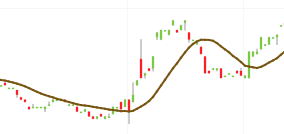Exponential moving average A type of moving average that is similar to a simple moving average, except that more weight is given to the latest data. It is more responsive to new information relative to the simple moving average. This responsiveness is one of the key factors of why this is the moving average of choice among many technical traders.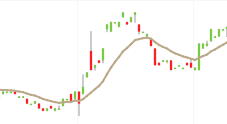Volume average Volume is the number of shares that trade over a given period of time, usually a day. The higher the volume, the more active the stock. Bollinger Bands The indicator shows two deviations from the moving average.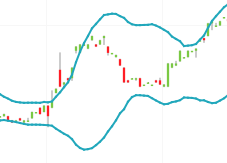RSI The Relative Strength Index indicator shows the overbought and oversold conditions of a stock. It ranges from 0 to 100. A stock is likely to be overbought once the RSI approaches the 70 level, meaning that it may be getting. Likewise, if the RSI approaches 30, it is an indication that the asset may be getting oversold and therefore likely to become undervalued.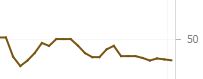MACD The Moving Average Convergence Divergence momentum indicator shows the relationship between two moving averages of prices. The most commonly used values are 12, 26, and 9 days, that is, MACD (12,26,9).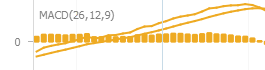Momentum The indicator compares the relation of the current price to the price in the past.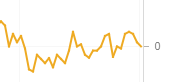Stochastic Fast The indicator calculates the ratio of two closing price statistics: the difference between the latest closing price and the lowest price in a given number of last days over the difference between the highest and lowest prices in the last days.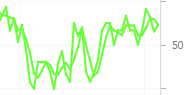Stochastic Slow The indicator calculates the simple moving average of the Stochastic Fast statistic across given periods.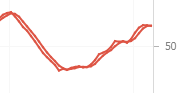VWAP The Volume-Weighted Average Price indicator provides the average price weighted by volume. It calculates the dollar value of all trading periods divided by the total trading volume for the current day.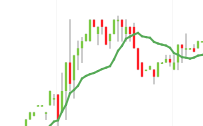PPO The Percentage Price Oscillator indicator that shows the relationship between two moving averages. The end result is a percentage that tells the trader where the short-term average is relative to the longer-term average. This allows a trader to use the PPO indicator to compare stocks with different prices more easily. For example, regardless of the stock's price, a PPO result of 20 means the short-term average is 20% above the long-term average.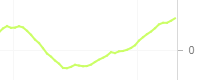Pivot Points The indicator is the average of the high, low and closing prices from the previous trading day.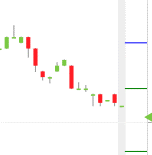Custom The application allows you to customize the existing indicators. For more details, see the procedures below.